# How To Calculate Mass From Force And Velocity: Several Approaches and Problem Examples

In previous articles, we’ve seen how to find mass with acceleration and force and without acceleration and force. So, in this post, we’ll look at how to calculate mass from force and velocity using several approaches and problems.

There are various approaches to finding mass, but Newton’s second law is the simplest. It helps you to find mass using force and velocity. Moreover, the centripetal force formula, work-energy theorem and kinematic equations of motion also help us find mass using the force and the velocity.

The mass, force and velocity are the words of physics that we also use in our day to day lives, and they are somehow interconnected.

Force is nothing but a physical effect that causes a change in an object or body’s state of motion. The mass, a fundamental property of every physical body, tells us how much matter that particular body contains. In effect, it behaves as the resistance offered by the body when it is forced to change its state either in terms of motion or position. When an object or body changes its position with time under the effect of force, this measurement refers to the object’s velocity.

Let’s take a look at each method of calculating mass using force and velocity one by one.

## How to calculate mass from force and velocity using Newton’s Second Law:

The second law of Newton can be expressed in various ways. The statement says that when a force acts on an objector particle, the force is equal to the change in momentum over time. The following is an equation that can be used to express the statement:

Here, the letter p can be used to signify the momentum of an object or particle. However, we know that it is the product of the object’s mass and velocity. As a result, it’s written mathematically as:

p = mv

We get the following when we substitute the above momentum equation in the force equation:

Now, mass increases only when an object’s velocity reaches the speed of light. However, this is not the case here. Because the velocity of the particle or object is not very high, i.e. close to the velocity of light, the mass of the object remains constant. As a result, we assume that only velocity, not mass, changes with time. As a result, the equation above can be represented as: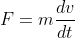……….(1)

Or,

F = ma……….(2)

Thus, in terms of force and velocity, the mass of an object can be calculated as follows: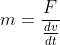……….(3)

Thus, from equation (3), if we know the force acting on the body and how velocity changes with time, it is easy to calculate mass using Newton’s second law.

## Problem: By applying a force of 25 N, the velocity of an object changes 5 m/s every second. What would be the mass of the object?

Given:

Force acting on object F = 25 N

Change in velocity dv = 5 m/s

Change in time dt = 1 s

To Find:

The mass of an object m =?

Solution:

The mass of an object:

∴ m = 5 kg

Thus, applying a force of 25 N on an object of mass 5 kg, its velocity changes 5 m/s every second.

## How to calculate mass from force and velocity using centripetal force:

When a body moves in a curved route, centripetal force exerts on it, which has an inward direction, or we might say towards the center. The centripetal force which acts on a body moving along a circular path of radius R is given by: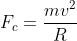……….(4)

Thus, the mass of an object moving on a circular path is given by: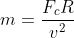……….(5)

Here, Fc is used for centripetal force.

This is how the mass of an object moving on a circular path can be calculated using centripetal force, velocity, and the radius of the path.

## Problem: Under the influence of 3 N centripetal force, a ball attached to the end of a string revolves in a horizontal circle with an angular speed of 5 rad s-1. What is the mass of a ball if the cord is 60 cm long?

Given:

Centripetal force Fc = 3 N

Angular speed ⍵ = 5 rad/s

Length of cord (radius of string) r  = 60 cm = 0.6 m

To find:

Mass of a ball m =?

Solution:

Before finding the mass of a ball, we find velocity.

Velocity in terms of angular velocity is given by:

v = ⍵r

∴ v = 5 X 0.6

∴ v = 3 m/s

Thus, mass of a ball:

∴ m = 0.2 kg = 200 gm

Thus, the mass of a ball is 200 gm.

## How to calculate mass from force and velocity using Third kinematic equation of motion:

The following is the third kinematic equation of motion:

It shows the relationship between the initial and final velocity. We may now apply Newton’s Second Law (equation (2)) to this equation and obtain:

v2 = u2 + 2(F/m)d

v2 – u2 = 2(F/m)d ……….(7)

Thus, using the third kinematic equation, the mass of an object can be calculated as follows: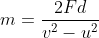……….(8)

If you know the distance (d) travelled by an object when its velocity v varies from its initial velocity u as a result of force F. In that instance, we can use the third kinematics equation of motion to calculate its mass.

## Problem: Assume an object is travelling at a velocity of 3 m/s. As a 20 N force is applied to the object, it moves 5 m at a velocity of 7 m/s. Determine the mass of an object.

Given:

Initial velocity of object u = 3 m/s

Finale velocity of object v = 7 m/s

Force applied to the object F = 20 N

Distance travelled by object under the effect of force d = 5 m

To find:

The mass of an object m =?

Solution:

Mass of the object is:

∴ m = 5 kg

Thus, the mass of an object is 5 kg.

## How to calculate mass from force and velocity using work energy theorem:

When the force is applied to an object, it moves a certain distance. As a result, according to the work energy theorem, the work done on the object to move this distance is equal to the kinetic energy gained by the object. In a nutshell, work is converted into energy. Putting it in mathematical terms:

W = K.E……….(9)

However, the work done on the object to move the distance d is as follows:

W = Fᐧd……….(10)

And the kinetic energy of an object with velocity v is: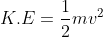……….(11)

From, equations (9), (10) and (11):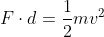……….(12)

Thus, using the work energy theorem, the mass of an object is given by: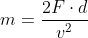……….(13)

Thus, we can argue that finding mass from the work energy theorem is a special case of finding mass from the third kinematic equation where the object’s initial velocity is zero, implying that it is initially thought to be at rest.

## Problem: Assume a box is lying on a horizontal surface. When pushed by a force of 60 N, it slides 15 m on the surface at a velocity of 30 m/s. Determine the mass of an object.

Given:

Force applied on box F = 60 N

Distance traveled by box under the effect of the force d = 15 m

Velocity of object during travelling that distance v = 30 m/s

To find:

The mass of a box m =?

Solution:

The mass of a box is:

∴ m = 2 kg

Thus, the mass of the box is 2 kg.

Scroll to Top